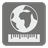Earth Distances
Simple
English

To describe a specific position on the surface of the Earth, we must rely on the geographic coordinate system. The geographic coordinate system is a method used to give every possible location on Earth to be specified by a set of numbers or letters. A common choice of coordinates is latitude and longitude. With this information we can calculate a distance between two points along a surface.

For simplicity’s sake, we will suppose that the Earth is a perfect sphere with a radius of 6,371 kilometers (it gives a mistake no more than 0.3%). You are given two point coordinates and you must find the shortest distance between these points on the surface of the Earth, measured along the surface of the Earth.

Coordinates are given as a string with the latitude and longitude separated by comma and/or whitespace. Latitude and longitude are represented in the follow format:

```    d°m′s″X
```

In this example, "d" is degrees, "m" is minutes, "s" is seconds as integers, while "X" is "N" (north) or "S" (south) for a latitude and "W" (west) or "E" (east) for a longitude.

The result should be given as a number in kilometers with a precision of ±0.1 (100 metres).

Input: Two arguments. Coordinates as strings (unicode).

Output: The distance as a number (int or float).

Example:

```distance(u"51°28′48″N 0°0′0″E", u"46°12′0″N, 6°9′0″E") == 739.2
distance(u"90°0′0″N 0°0′0″E", u"90°0′0″S, 0°0′0″W") == 20015.1```

How it is used: The concepts presented in this mission are the exact sorts of concepts used in navigational software, enabling a ship or plane to understand where it is, where it must go and how far it has gone. Along the same vein, Global Positioning Satellites use similar principles to provide pinpoint accurate locations to GPS receivers for use in navigation.

Precondition: Correct Coordinates.